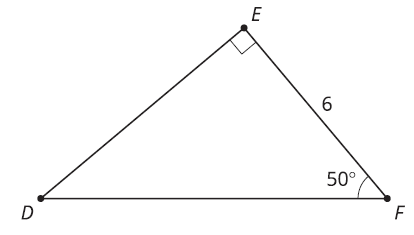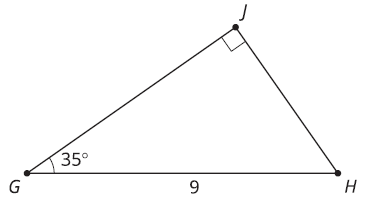# G.4 - 6 Using Trigonometric Ratios to Find Missing Side Lengths

Topic:
Ratios
Here's the complete directions for finding the side length of a right triangle using sine, cosine, or tangent.
1. Label the side you're trying to find with x.
2. Use opposite, adjacent, or hypotenuse to label the side you know & the side you want to find.
3. Choose the trig ratio that involves the two sides you just labeled.
4. Set up an equation using the trig ratio you choose by filling in the angle and side length you know and x for the side you don't know.
5. Solve the equation.Let's try all 5 steps to find the length of DF.Let's try all 5 steps to find the length of DE.Let's check our answers for DF and DE using the pythagorean theorem.Find the length of side JH.Find the length of side GJ.Check your answers for JH and GJ using the pythagorean theorem.